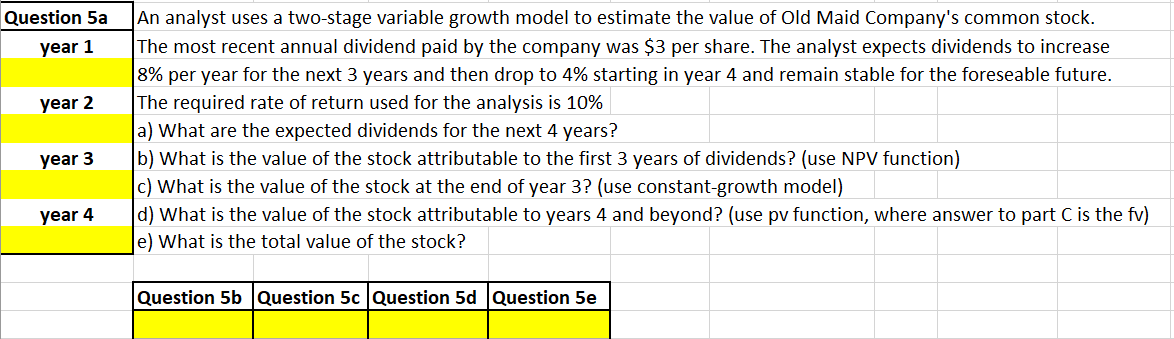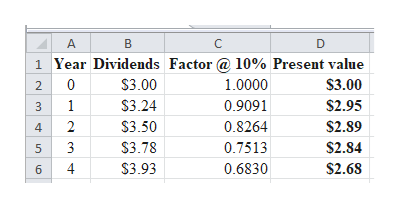# An analyst uses a two-stage variable growth model to estimate the value of Old Maid Company's common stock.The most recent annual dividend paid by the company was \$3 per share. The analyst expects dividends to increase8% per year for the next 3 years and then drop to 4% starting in year 4 and remain stable for the foreseable future.The required rate of return used for the analysis is 10%a) What are the expected dividends for the next 4 years?b) What is the value of the stock attributable to the first 3 years of dividends? (use NPV function)c) What is the value of the stock at the end of year 3? (use constant-growth model)d) What is the value of the stock attributable to years 4 and beyond? (use pv function, where answer to part C is the fv)e) What is the total value of the stock?Question 5ayear 1year 2year 3year 4Question 5b Question 5c Question 5d Question 5e

Question
119 views

Please show how to do this in Excel.  Thank you.help_outlineImage TranscriptioncloseAn analyst uses a two-stage variable growth model to estimate the value of Old Maid Company's common stock. The most recent annual dividend paid by the company was \$3 per share. The analyst expects dividends to increase 8% per year for the next 3 years and then drop to 4% starting in year 4 and remain stable for the foreseable future. The required rate of return used for the analysis is 10% a) What are the expected dividends for the next 4 years? b) What is the value of the stock attributable to the first 3 years of dividends? (use NPV function) c) What is the value of the stock at the end of year 3? (use constant-growth model) d) What is the value of the stock attributable to years 4 and beyond? (use pv function, where answer to part C is the fv) e) What is the total value of the stock? Question 5a year 1 year 2 year 3 year 4 Question 5b Question 5c Question 5d Question 5e fullscreen
check_circle

Step 1

“Hi, as per our policy we will answer the first question. Kindly repost the remaining questions separately in order to be answered”

Step 2

a)

Computation of expected dividends for the next 4 years:

Excel sp...help_outlineImage TranscriptioncloseD 1 Year Dividends Factor @ 10% Present value \$3.00 1.0000 \$3.00 2 0 \$3.24 0.9091 \$2.95 2 \$3.50 0.8264 \$2.89 4 3 \$3.78 0.7513 \$2.84 4 \$3.93 0.6830 \$2.68 6. fullscreen

### Want to see the full answer?

See Solution

#### Want to see this answer and more?

Solutions are written by subject experts who are available 24/7. Questions are typically answered within 1 hour.*

See Solution
*Response times may vary by subject and question.
Tagged in